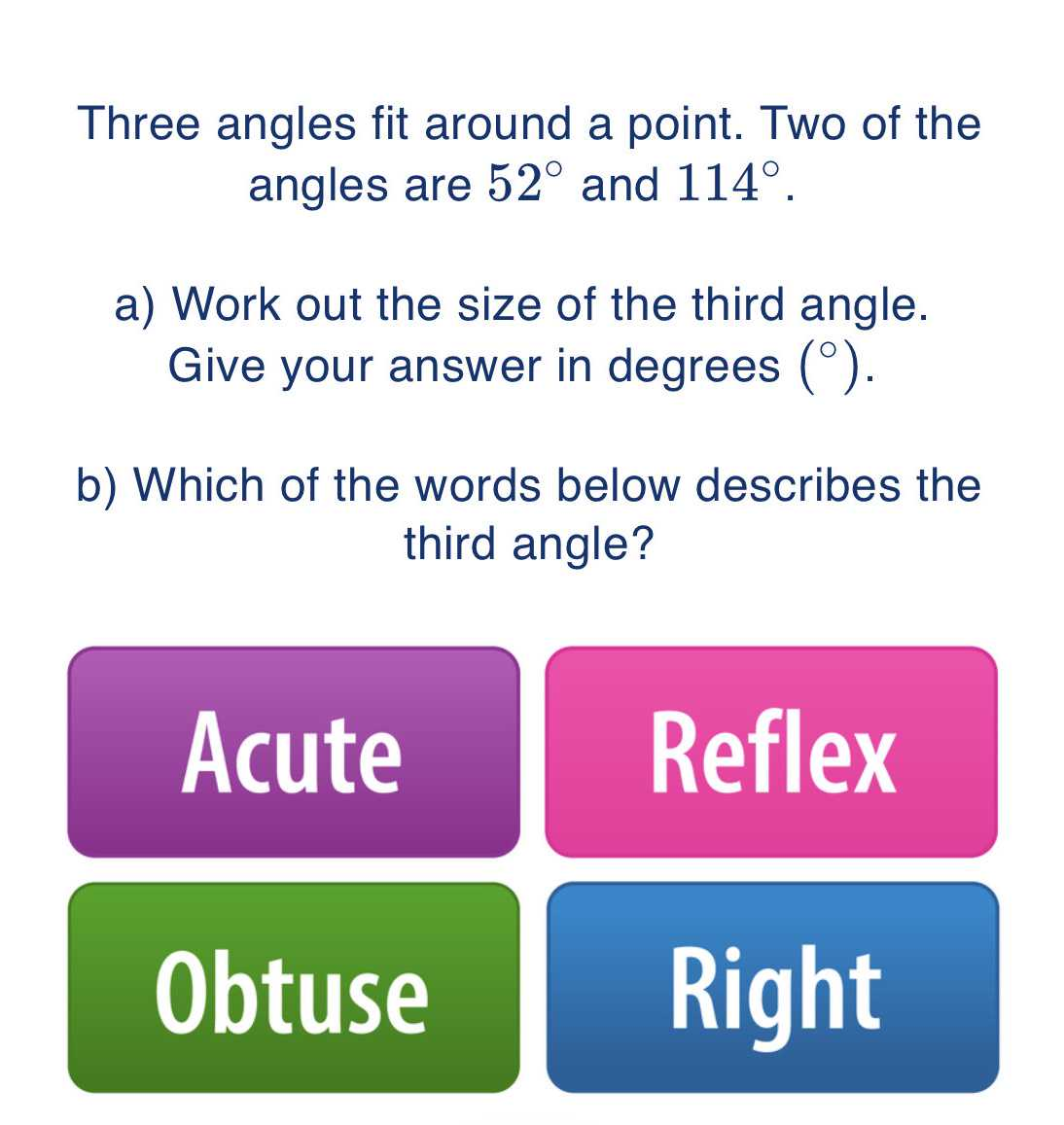### Still have math questions?

Trigonometry
QuestionThree angles fit around a point. Two of the angles are $$52 ^ { \circ }$$ and $$114 ^ { \circ }$$ .

a) Work out the size of the third angle. Give your answer in degrees $$( ^ { \circ } )$$ .

b) Which of the words below describes the third angle?

A. Acute

B. Reflex

C. Obtuse

D. Right

Third angle is 194$$°$$